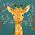## Heart's Proportion Problems

1.) You estimate that you can do 12 math problems in 45 mins. How long should it take you to do 20 math problems?

Let x be the amount of minutes it took you to do 20 math problems
Word ratio, ratio table, ratio
It will take you 75 minutes in order to finish the 20 Math problems.

2.) The ratio of an object's weight on Earth to its weight on the Moon is 6:1. The first person to walk on the moon is Neil Armstrong. He weighed 165 pounds on Earth. How much did he weighed on the moon?

Let x be the amount of pounds Neil Armstrong's weight on the moon.
Word ratio, ratio table, ratio
Neil Armstrong will weigh 27.5 pounds on the moon.

At the copy shop, 18 copies cost \$1.08. At that rate, how much will 40 copied cost?

2:38 PM

#### 2 comments :

1.The answer for the question is..
Let "x" be the cost of 40 copies
Steps : word ratio , ratio table , find x

Copies Cost 18x=1.08x40
18 \$1.08 18x /18=43.2/18
40 x x=2.4

40 copies will cost \$2.4

2.